Please wait while the marmots are preparing the hot chocolate…
# {image-full darkened /black-bg /no-status title-slide} // commentaire
- - - (best viewed with firefox (maths)) ## What's on the menu today? {#overview overview} - Probabilities and normal distribution - Gaussian mixture models - EM and learning mixture models from data - Choosing the number of components - Dirichlet processes - Examples? # Just Some Reminders (Probably Almost Surely) ## Probability Rules, Measure Theory @svg: floatrightbr,aa proba/potato-xandy.svg 245px 135px @svg: floatrightbr,bb proba/potato-xsumy.svg 245px 285px - Product rule{prod} - $p(X, Y) = p(X|Y) \, p(Y)$ = $p(Y|X) \, p(X)$

- Marginalization, Sum rule{sum} - $p(X) = \sum_{Y \in \mathcal{Y}} p(X,Y)$ - $p(X) = \int_{Y \in \mathcal{Y}} p(X,Y)$ - Bayes rule{bayes} - $p(Y|X) = \frac{p(X|Y) \, p(Y)}{p(X)}$ - $p(X) = \sum_{Y\in \mathcal{Y}} p(X|Y) \, p(Y)${denom} - @anim: .aa + .prod | .bb + .sum | .app | .bayes | .denom # Gaussian/Normal Distribution ## Gaussian/Normal Distribution (1D) @svg: gaussian/normal-distribution-stddev.svg 800px 500px {floatrightbr fullabs} ## Gaussian/Normal Distribution (1D) @svg: gaussian/normal-distribution-stddev.svg 200px 200px {floatrightbr} - Normal Distribution or Gaussian Distribution{bb} - $\mathcal{N}(x|\mu,\sigma^2) = \frac{1}{\sqrt{2\pi \sigma^2}} exp(-\frac{(x-\mu)^2}{2 \sigma^2})$ - Is-a probability density{bbb} - $\int_{-\infty}^{+\infty} \mathcal{N}(x|\mu,\sigma^2) dx = 1${bbb} - $\mathcal{N}(x|\mu,\sigma^2) > 0${bbb} - Parameters{cc} - $\mu$: mean, $E[X] = \mu$ - $\sigma^2$: variance, $E[(X -E[X])^2 ] = \sigma^2$ - @anim: .bb |.bbb |.cc ## Multivariate Normal Distribution - D-dimensional space: $x = \{x\_1, ..., x_D\}$ - Probability distribution{slide} - $\mathcal{N}(x|\mu,\Sigma) = \frac{1}{\sqrt{(2\pi)^D \|\Sigma\|}}\; exp(-\frac{(x-\mu)^T\Sigma^{-1}(x-\mu)}{2})$ - $\Sigma$: covariance matrix - @anim: .hasSVG @svg: floatleft gaussian/multivariate-normal.svg 800px 250px # Gaussian Mixture Models ## Gaussian Mixture Model (1D) - Density: $p(x | \theta) = \sum_{k=1}^K w\_k \;\mathcal{N}(x|\mu\_k,\sigma\_k^2)$ {slide} - Parameters: $\theta = (w\_k, \mu\_k, {\sigma\_k}^2)_{k=1..K}$ {slide} @svg: gmm/two-gaussians-gmm.svg 800px 400px {unpadtop50} ## Sampling from a 2D GMM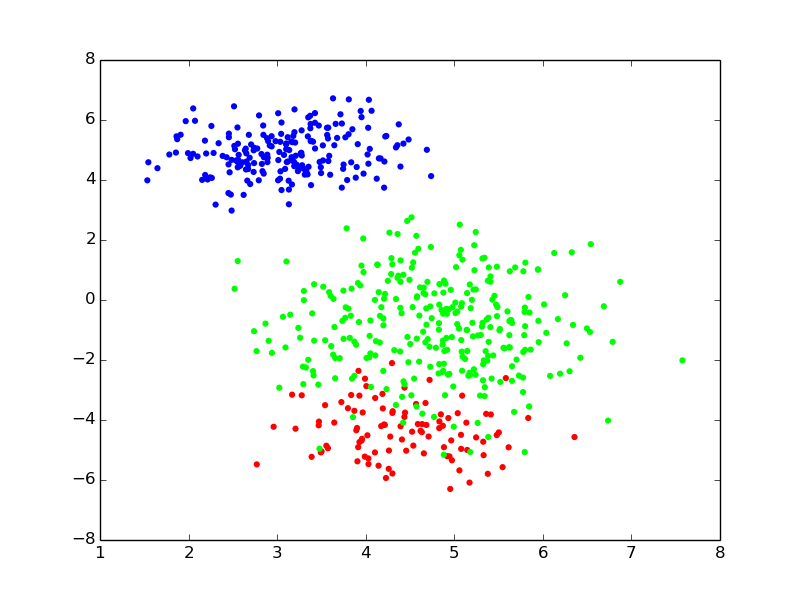-  {no} - Density: $p(x | \theta) = \sum_{k=1}^K w\_k \;\mathcal{N}(x|\mu\_k,\Sigma\_k)$ - Parameters: $\theta = (w\_k, \mu\_k, \Sigma\_k)_{k=1..K}$ - For each point $i$ to be generated {clearboth} - draw a **component index** (i.e., a color): $z_i \sim \operatorname{Categorical}(w)$ - draw its **position** from the component: $x\_i \sim \mathcal{N}(x|\mu\_{z\_i},\Sigma\_{z_i})$ ## Only Positions are Observed {pfree}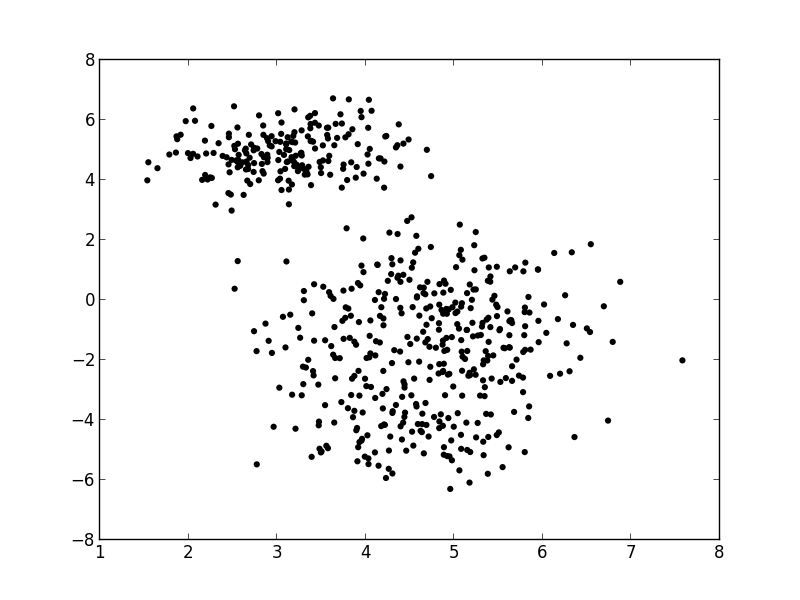- For each point $i$ {clearboth} - draw a component/color: $z_i \sim \operatorname{Categorical}(w)$ - draw a position: $x\_i \sim \mathcal{N}(x|\mu\_{z\_i},\Sigma\_{z_i})$ - Finding $z$ seems impossible,
finding the “best” $\theta$ might be feasible? {slide} ## GMM: Probabilistic Graphical Model @svg: graphical-models-dpgmm/dp-finite-no-prior.svg 800px 200px - Renamed - $w$ → $\beta$ (vector of component weights) - $(\mu\_k, \Sigma\_k)$ → $\phi_k$ (parameters of component $k$) - Reminder: for each point $i$ {clearboth} - draw a component/color: $z_i \sim \operatorname{Categorical}(\beta)$ - draw a position: $x\_i \sim \mathcal{N}(x|\phi\_{z\_i})$ # Learning/Inference
finding the best $\theta$ ## Maximum Likehood in GMM - From a set of observed points $x = \\{x\_i\\}\_{i=1..N}$ - Maximize the likelihood of the model {libyli} - NB: $\mathcal{L}(\theta | x) = p(x | \theta)$ - NB: $\operatorname{arg max}\_{\theta}\mathcal{L}(\theta | x) = \operatorname{arg max}\_{\theta}\log \mathcal{L}(\theta | x)$ - indep: $\log p(x | \theta) = \log \prod\_{i=1}^N p(x\_i | \theta) = \sum\_{i=1..N} \log p(x\_i | \theta)$ - mixture: $\log p(x | \theta) = \sum\_{i=1}^N \log \sum\_{k=1}^K w\_k \; \mathcal{N}(x\_i | \phi\_k)$ - No closed form expression → need to approximate{slide} # Expectation
Maximization ## Expectation-Maximization - Approximate inference by local optimization - converges to local optimum - needs a “good” initialization - Handling parameters and latent variables differently {slide} - single (point) estimate of the parameters $\theta$ - distribution estimate of the latent variables $z$ - Two-step iterative algorithm {libyli slide} - init: select a value for the parameters $\theta^0$ - E step: - compute the distribution over the latent variables - i.e., $\forall i,k \;\; p(z\_i = k | \theta^t)$ - these probabilities are called the “responsibilities” - M step: - find the best parameters $\theta$ given the responsibilities - i.e., $\theta^{t+1} = \operatorname{arg max}\_{\theta}$ ## EM iterations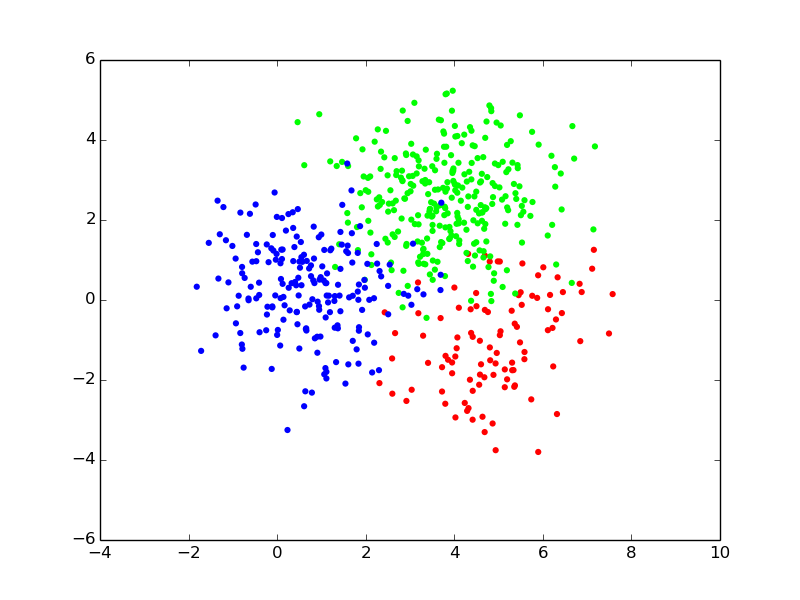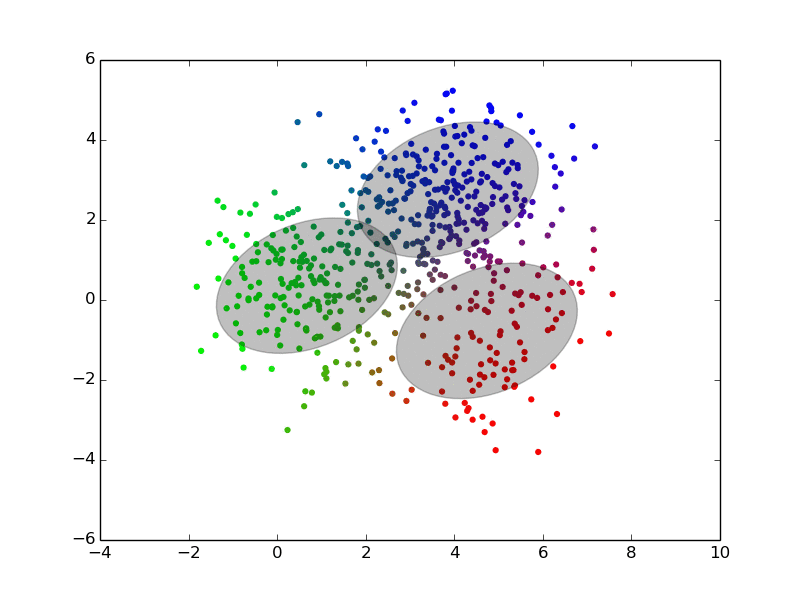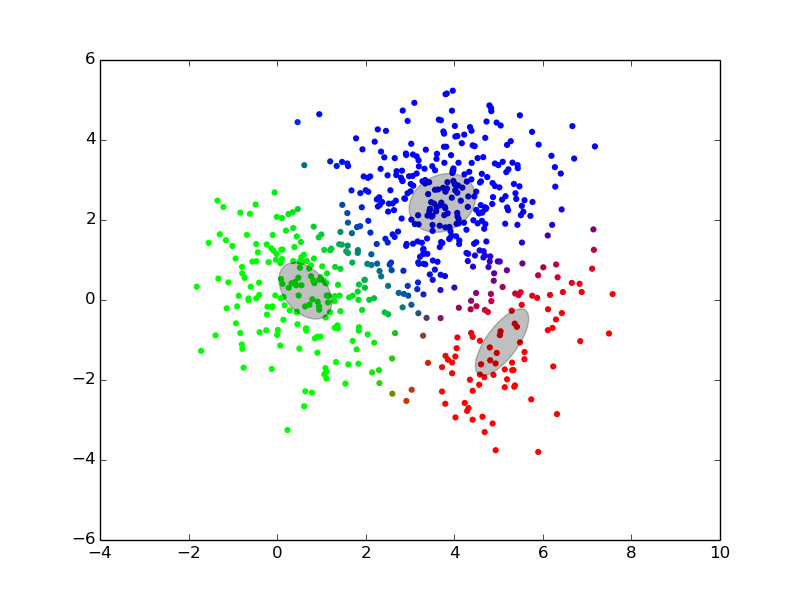## EM iterations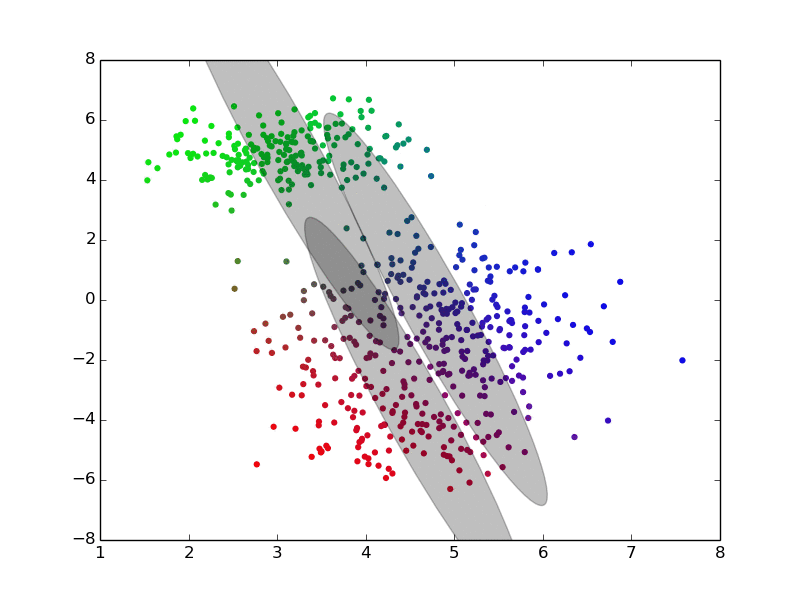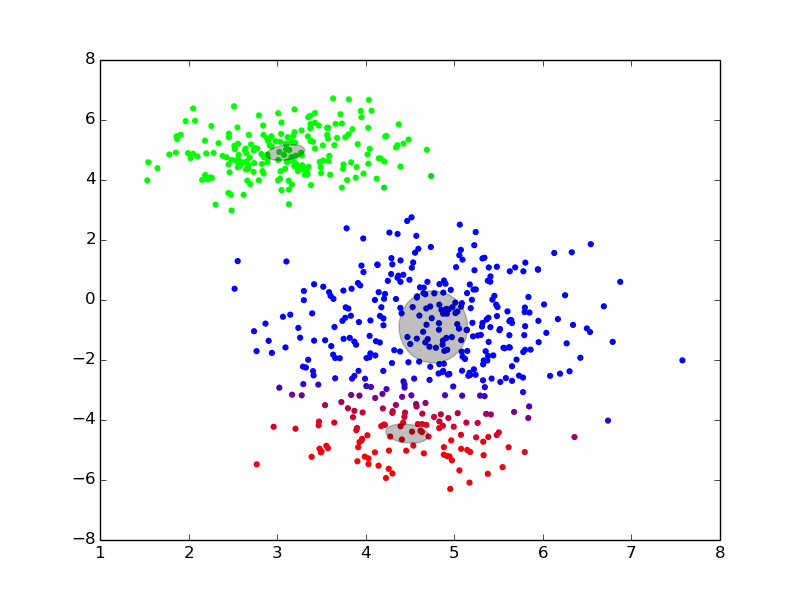## EM iterations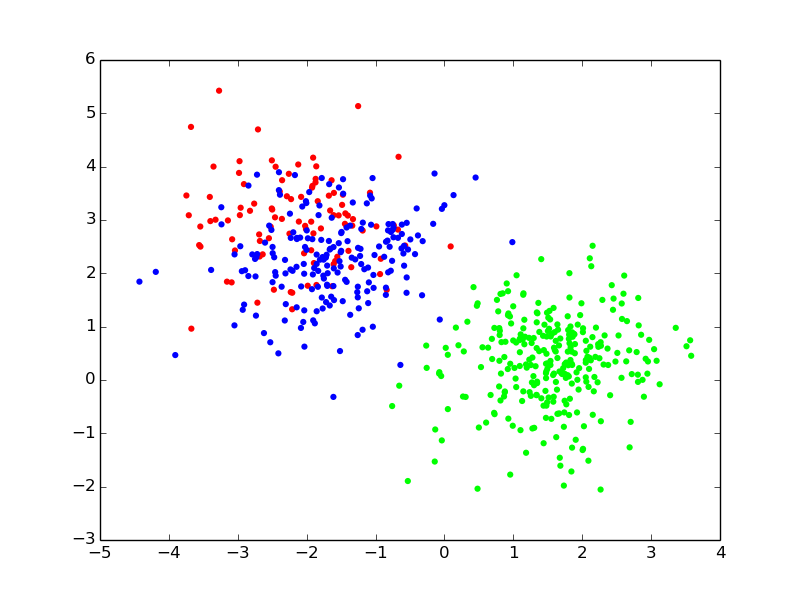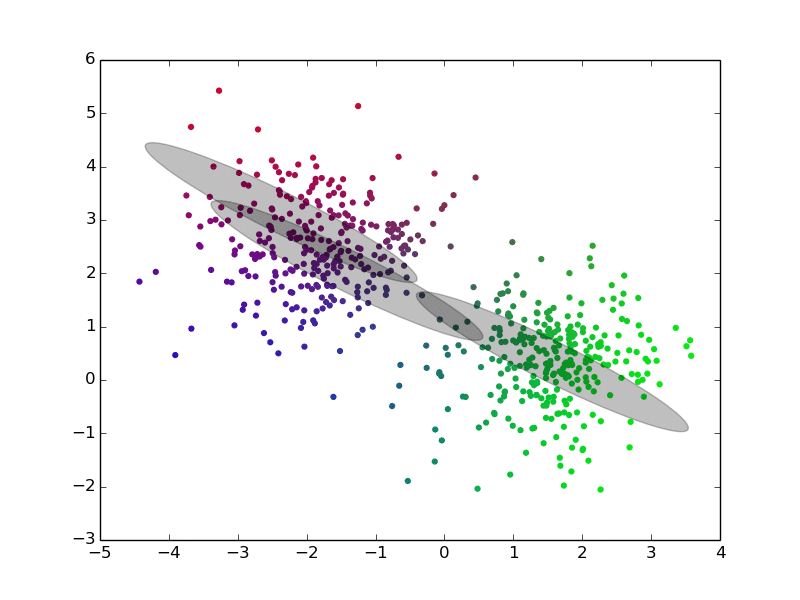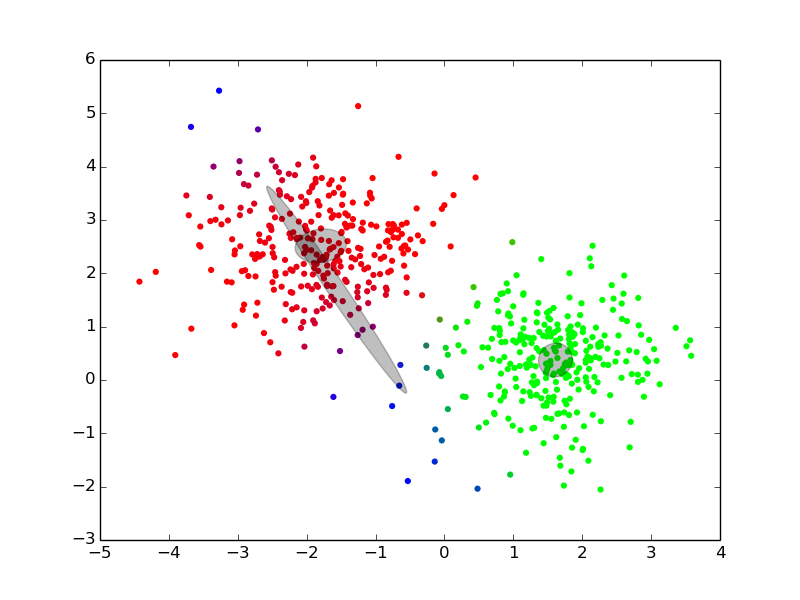## EM, Gibbs Sampling, Variational Inference {densetitle} @svg: media/em-gibbs-variational.svg 800px 500px {unpademgibbsvariational} - @anim: #header, #em | #eme | #emm | #gibbs | #gibbsloop | #cgibbs | #variational # Beyond EM, using prior ## Prior on GMM @svg: graphical-models-dpgmm/dp-finite-no-prior.svg 200px 200px {floatright light} @svg: graphical-models-dpgmm/dp-finite.svg 200px 200px {floatright other} - It's all about prior - Intution in EM - disappearing component - “minimal” weight - “minimal” variance -   {no notslide clearboth} - We virtually add a few observations to each component {slide} - $\alpha$: causes the weights $\beta$ to never be zero {slide} - Dirichlet distribution: $\beta \sim \operatorname{Dirichlet}(\alpha)$ - $H$: adds some regularization on the variances $\Sigma_k$ {slide} # … unfold @svg: graphical-models-dpgmm/dp-finite.svg 200px 200px {other} @svg: graphical-models-dpgmm/dp-finite-split.svg 200px 200px {other} # Dirichlet Processes ## From GMM to DPGMM @svg: graphical-models-dpgmm/dp-finite-split.svg 200px 200px {floatright light} - Plain GMM - the number of component $K$ needs to be know - need to try multiple ones and do model selection - (prior are not handled by EM) - {no clearboth} @svg: graphical-models-dpgmm/dp-split.svg 200px 200px {floatright other clearboth} - Dirichlet Process GMM - a GMM with an infinity of components - with a proper prior - cannot use EM for inference (infinite vectors) # What is a Dirichlet Process, finally ## Dirichlet Process @svg: graphical-models-dpgmm/dp-split.svg 200px 200px {floatright} - What? {slide} - a distribution over distributions - a prior over distributions - two parameters - a scalar $\alpha$, the “concentration” - a “base” distribution $H$ (any type) - a draw from a DP is a countably infinite sum of Diracs - Related formulations {libyli slide} - Definition: complicated :) - Stick breaking process (GEM) - as shown on the graphical model - e.g., a prior on the values of the weights - Chinese Restaurant Process (CRP) - how to generate the $z_i$, one by one, in sequence - Polya Urn ## Definition Example (Wikipedia) @svg: graphical-models-dpgmm/example-wikipedia.svg 500px 400px {floatright} Draws from the Dirichlet process DP(N(0,1), alpha). Each row uses a different alpha: 1, 10, 100 and 1000. A row contains 3 repetitions of the same experiment. ## Stick breaking process (GEM) @svg: graphical-models-dpgmm/dp-split.svg 200px 200px {floatright} - Each “atom” drawn from $H$ - Infinite vector drawn from a GEM process - $p\_1 \sim Beta(1, \alpha)$ ; $\beta\_1 = p\_1$ - $p\_2 \sim Beta(1, \alpha)$ ; $\beta\_2 = (1 - \beta\_1) * p\_2$ - $p\_3 \sim Beta(1, \alpha)$ ; $\beta\_3 = (1 - \beta\_1 - \beta\_2) * p\_3$ - ... - and $\forall k \;\; \Phi_k \sim H$ - Denoted $\beta \sim GEM(\alpha)$ - then $G = \sum^\infty\_{k=1} \beta\_k \delta\_{\Phi\_k}$ is a draw from $DP(H, \alpha)$ - (GEM for Griths, Engen and McCloskey) # Polya Urn? ## Chinese Restaurant Process (CRP) - Gibbs sampling friendly - easy to get $p(z\_i | z^{-i}, ...)$ - $p(z\_i | z^{-i}) = ...$

/ will be replaced by the authorwill be replaced by the title